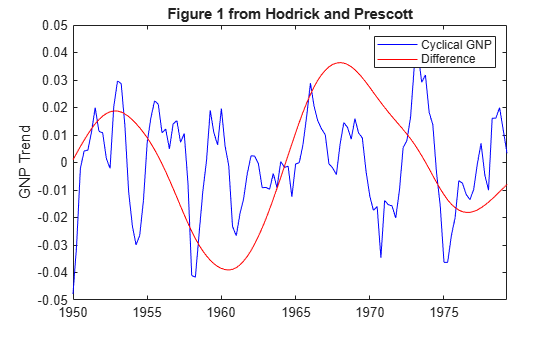# Use Hodrick-Prescott Filter to Reproduce Original Result

This example shows how to use the Hodrick-Prescott filter to decompose a time series.

The Hodrick-Prescott filter specialized filter for trend and business cycle estimation. The filter separates a time series into trend and cyclical components (no seasonal components), the latter of which is often of interest to business cycle analysts.

Suppose a time series ${\mathit{y}}_{\mathit{t}}$ can be additively decomposed into a trend ${\tau }_{\mathit{t}}$ and business cycle component ${\mathit{c}}_{\mathit{t}}$, such that, for $\mathit{t}=1,...,\mathit{T}$,

`${y}_{t}={\tau }_{t}+{c}_{t}.$`

The objective function for the filter is

`$\sum _{t=1}^{T}{c}_{t}^{2}+\lambda \sum _{t=2}^{T-1}\left(\left({\tau }_{t+1}-{\tau }_{t}\right)-\left({\tau }_{t}-{\tau }_{t-1}\right){\right)}^{2}.$`

The programming problem is to minimize the objective over all trends components ${\tau }_{1},...,{\tau }_{\mathit{T}}$. The hyperparameter $\lambda$ is a nonnegative smoothing parameter that penalizes the objective for large secod-order differences of the trend component.

The conceptual basis for this programming problem is that the first sum minimizes the difference between the data and its trend component (which is the cyclical component) and the second sum minimizes the second-order difference of the trend component, which is analogous to minimization of the second derivative of the trend component. The programmuing problem is equivalent to that of a cubic spline smoother.

### Use Hodrick-Prescott Filter to Analyze GNP Cyclicality

Using data similar to the data found in Hodrick and Prescott , plot the cyclical component of GNP. This result should coincide with the results in the paper. However, since the GNP data here and in the paper are both adjusted for seasonal variations with conversion from nominal to real values, differences can be expected due to differences in the sources for the pair of adjustments. Note that our data comes from the St. Louis Federal Reserve FRED database , which was downloaded with the Datafeed Toolbox™.

```load Data_GNP % Change the following two lines to try alternative periods startdate = datetime(1950,1,1); enddate = datetime(1979,4,1); DTT = DataTimeTable(startdate:enddate,:); DTT.GNPRLog = log(DTT.GNPR); figure plot(DTT.Time,DTT.GNPRLog) title("Gross National Product (Log)")```Filter the series for each smoothing parameter value $\lambda$ = 400, 1600, 6400, and $\infty$. The infinite smoothing parameter detrends the data.

```[TTbl4,CTbl4] = hpfilter(DTT,Smoothing=400,DataVariables="GNPRLog"); [TTbl16,CTbl16] = hpfilter(DTT,Smoothing=1600,DataVariables="GNPRLog"); [TTbl64,CTbl64] = hpfilter(DTT,Smoothing=6400,DataVariables="GNPRLog"); [TTblInf,CTblInf] = hpfilter(DTT,Smoothing=Inf,DataVariables="GNPRLog");```

### Plot Cyclical GNP and Its Relationship With Long-Term Trend

Generate Figure 1 from Hodrick and Prescott  by setting the numeric slider interactive control for $\lambda$ to 1600.

```lambda =1600; [~,CTbl] = hpfilter(DTT,lambda,DataVariables="GNPRLog"); plot(DTT.Time,CTbl.GNPRLog,"b"); hold all plot(DTT.Time,CTblInf.GNPRLog - CTbl.GNPRLog,"r"); title("Figure 1 from Hodrick and Prescott"); ylabel("GNP Trend"); legend(["Cyclical GNP" "Difference"]); hold off```The blue line is the cyclical component with smoothing parameter 1600 and the red line is the difference with respect to the detrended cyclical component. The difference is smooth enough to suggest that the choice of smoothing parameter is appropriate.

You can use the numeric slider control to tune the filter interactively.

### Statistical Tests on Cyclical GNP

Reconstruct Table 1 from Hodrick and Prescott . With the cyclical components, compute standard deviations, autocorrelations for lags 1 to 10, and perform a Dickey-Fuller unit root test to assess non-stationarity.

```ACFTbl4 = autocorr(CTbl4,NumLags=10,DataVariable="GNPRLog"); ACFTbl16 = autocorr(CTbl16,NumLags=10,DataVariable="GNPRLog"); ACFTbl64 = autocorr(CTbl64,NumLags=10,DataVariable="GNPRLog"); ACFTblInf = autocorr(CTblInf,NumLags=10,DataVariable="GNPRLog"); [StatTbl4] = adftest(CTbl4,Model="ARD"); [StatTbl16] = adftest(CTbl16,Model="ARD"); [StatTbl64] = adftest(CTbl64,Model="ARD"); [StatTblInf] = adftest(CTblInf,Model="ARD"); displayResults(CTbl4,CTbl16,CTbl64,CTblInf, ... ACFTbl4,ACFTbl16,ACFTbl64,ACFTblInf, ... StatTbl4,StatTbl16,StatTbl64,StatTblInf);```
```Table 1 from Hodrick and Prescott Reference Smoothing Parameter 400 1600 6400 Infinity Std. Dev. 1.52 1.75 2.06 3.11 Autocorrelations 1 0.74 0.78 0.82 0.92 2 0.38 0.47 0.57 0.81 3 0.05 0.17 0.33 0.70 4 -0.21 -0.07 0.12 0.59 5 -0.36 -0.24 -0.03 0.50 6 -0.39 -0.30 -0.10 0.44 7 -0.35 -0.31 -0.13 0.39 8 -0.28 -0.29 -0.15 0.35 9 -0.22 -0.26 -0.15 0.31 10 -0.19 -0.25 -0.17 0.26 Unit Root -4.35 -4.13 -3.79 -2.28 Reject H0 1 1 1 0 ```

As shown in , as $\lambda$ increases, standard deviations increase, autocorrelations increase over longer lags, and the unit root hypothesis is rejected for all but the detrended case. These results imply that any of the cyclical series with finite smoothing is effectively stationary.

### Local Function

```function displayResults(gnpcycle4,gnpcycle16,gnpcycle64,gnpcycleinf,... gnpacf4,gnpacf16,gnpacf64,gnpacfinf,... gnptest4,gnptest16,gnptest64,gnptestinf) % DISPLAYRESULTS Display cyclical GNP test results in tabular form fprintf(1,'Table 1 from Hodrick and Prescott Reference\n'); fprintf(1,' %10s %s\n',' ','Smoothing Parameter'); fprintf(1,' %10s %10s %10s %10s %10s\n',' ','400','1600','6400','Infinity'); fprintf(1,' %-10s %10.2f %10.2f %10.2f %10.2f\n','Std. Dev.', ... 100*std(gnpcycle4.GNPRLog),100*std(gnpcycle16.GNPRLog), ... 100*std(gnpcycle64.GNPRLog),100*std(gnpcycleinf.GNPRLog)); fprintf(1,' Autocorrelations\n'); for i = 2:11 fprintf(1,' %10g %10.2f %10.2f %10.2f %10.2f\n',(i-1), ... gnpacf4.ACF(i),gnpacf16.ACF(i),gnpacf64.ACF(i),gnpacfinf.ACF(i)) end fprintf(1,' %-10s %10.2f %10.2f %10.2f %10.2f\n','Unit Root', ... gnptest4.stat,gnptest16.stat,gnptest64.stat,gnptestinf.stat); fprintf(1,' %-10s %10d %10d %10d %10d\n','Reject H0',... gnptest4.h,gnptest16.h,gnptest64.h,gnptestinf.h); end```

 Hodrick, Robert J., and Edward C. Prescott. "Postwar U.S. Business Cycles: An Empirical Investigation." Journal of Money, Credit and Banking 29, no. 1 (February 1997): 1–16. https://doi.org/10.2307/2953682.

 U.S. Federal Reserve Economic Data (FRED), Federal Reserve Bank of St. Louis, `https://fred.stlouisfed.org/`.